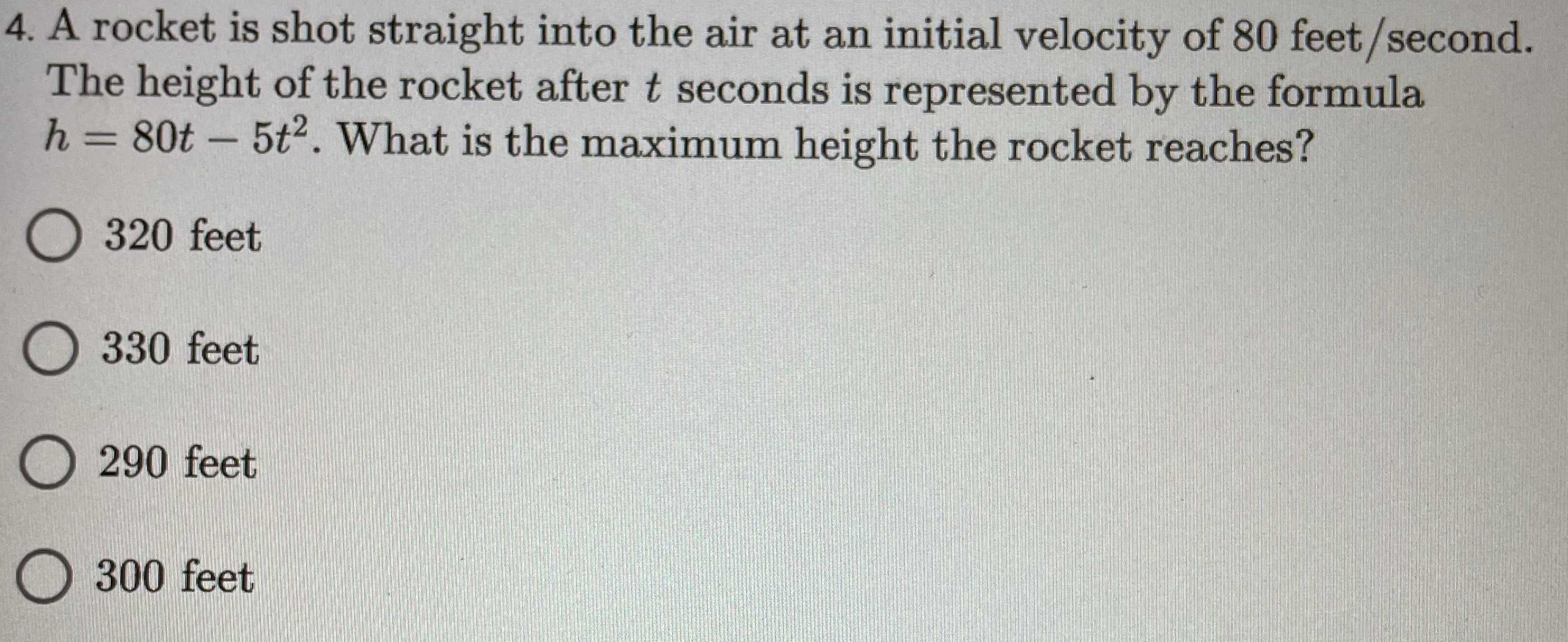### ¿Todavía tienes preguntas de matemáticas?

Pregunte a nuestros tutores expertos
Algebra
Pregunta4. A rocket is shot straight into the air at an initial velocity of $$80$$ feet/second. The height of the rocket after $$t$$ seconds is represented by the formula

$$h = 80 t - 5 t ^ { 2 } .$$ What is the maximum height the rocket reaches?

$$320$$ feet

$$330$$ feet

$$290$$ feet

$$300$$ feet